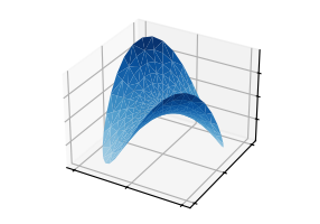# mpl_toolkits.mplot3d.axes3d.Axes3D.plot_trisurf#

Axes3D.plot_trisurf(*args, color=None, norm=None, vmin=None, vmax=None, lightsource=None, **kwargs)[source]#

Plot a triangulated surface.

The (optional) triangulation can be specified in one of two ways; either:

```plot_trisurf(triangulation, ...)
```

where triangulation is a `Triangulation` object, or:

```plot_trisurf(X, Y, ...)
plot_trisurf(X, Y, triangles, ...)
plot_trisurf(X, Y, triangles=triangles, ...)
```

in which case a Triangulation object will be created. See `Triangulation` for an explanation of these possibilities.

The remaining arguments are:

```plot_trisurf(..., Z)
```

where Z is the array of values to contour, one per point in the triangulation.

Parameters:
X, Y, Zarray-like

Data values as 1D arrays.

color

Color of the surface patches.

cmap

A colormap for the surface patches.

normNormalize

An instance of Normalize to map values to colors.

vmin, vmaxfloat, default: None

Minimum and maximum value to map.

Whether to shade the facecolors. Shading is always disabled when cmap is specified.

lightsource`LightSource`

The lightsource to use when shade is True.

**kwargs

All other keyword arguments are passed on to `Poly3DCollection`

Examples

## Examples using `mpl_toolkits.mplot3d.axes3d.Axes3D.plot_trisurf`#plot_trisurf(x, y, z)

plot_trisurf(x, y, z)